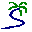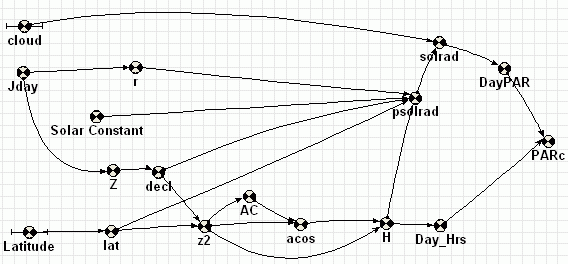# Model entry

Solar radiation incident at the earth's surface - Model catalogue - Simulistics.com

 Search Simulistics Model catalogue Listed by keyword Listed by ID Listed by title Listed by date added

# Solar radiation incident at the earth's surface

Simile version : 4.0
Keywords : Solar radiation ; Ecophysiology ;

## Description

This model estimates the daily solar radiation incident at the earth's surface a given latitude and after passing through an atmosphere of given opacity (the cloudiness parameter in the model). Particularly it estimates:

• day length (hours)
• potential insolation on a horizontal plane (J m-2day-1)
• daily Photon Flux Density (PFD) sometimes refered to as, now deprecated, Photosynthetically Active Radiation (PAR)

The equations here were taken from the DNDC model (Li et al., 1992)

## Files

### Model file

Click on the icon to download the model file. (You will need Simile to examine and run the model. A free evaluation version is available from the products page.)

Some browsers may attempt to display the model file, rather than open it in Simile; in this case, use the browser back button to return to this page, and use the context menu (invoked by right-clicking on the link) to save the target file to disk.SolarRadiationDNDCP.sml

## Diagram## Equations

```Equations in climateP1

Variable   AC
AC = TA=abs(z2),if TA<0.7 then 1.571-atan(TA/(1-TA^2)^0.5)else atan((1-TA^2)^0.5/TA)

Variable   DayPAR : PAR (moles/m^2/day)

Variable   Day_Hrs : Length of day (hr)
Day_Hrs = 2*(H*24)/(2*3.1416)

Variable   H : Sun elevation angle at noon (radians)
H = if z2>=1 then 0 elseif z2<= -1 then pi()else acos

Variable   Jday : Day of Year
Jday = time()

Variable   PARc : Daily instantaneous PAR (umol m^-2 s^-1)
PARc = DayPAR/(Day_Hrs*0.0036)

Variable   Solar Constant : Solar constant (W m^2)
Solar Constant = 1367.0
solar max Lean 1991 Rev. Geophysics 29

Variable   Z
Z = 0.398*sin(4.869+0.0172*Jday+0.0334*sin(6.224+0.0172*Jday))

Variable   acos
acos = if z2<0 then 3.1416-AC else AC

Variable   decl : Declination of the sun (radians)
decl = if abs(Z)<0.7 then atan(Z/(1-Z^2)^0.5)else pi()/2-atan((1-Z^2)^0.5/Z)

lat = l=Latitude*pi()/1.8E+02,if abs(l*1.0)>=pi()/2 then sgn(l*1.0)*(pi()/2-0.01)else l
abs(lat) < 90 degrees

Variable   psolrad : potential insolation on a horizontal plane, J/m^2/day
Where:
isc=Solar Constant

Variable   r : radius vector of the sun
r = 1.0-0.0167*cos(0.0172*(Jday-3))

||